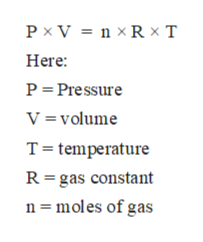# Calculate the final Kelvin temperature of sulfur dioxide if the gas at 20 degrees Celsius and 0.450 atm is heated until the pressure is 0.750 atm.

Question
34 views

Calculate the final Kelvin temperature of sulfur dioxide if the gas at 20 degrees Celsius and 0.450 atm is heated until the pressure is 0.750 atm.

check_circle

star
star
star
star
star
1 Rating
Step 1

All matter can be classified as solid, liquid and gases mainly. In these physical states of matter, the solid state is composed of particles with least intermolecular distance and strongest intermolecular force of attraction. On the contrary, the gaseous state of matter is composed of particles with largest intermolecular distance and weakest intermolecular force of attraction.

Step 2

The gaseous state is the state of matter with least intermolecular force of attraction between molecules and highest kinetic energy. The standard properties of gases like Pressure, volume, and temperature can be calculated with the help of ideal gas equation.

The ideal gas equation can be written as:help_outlineImage TranscriptionclosePx V = nxR - T Here P Pressure V volume T temperature R gas constant n moles of gas fullscreen
Step 3

Joseph Gay-Lussac law states that the pressure is directly proportional to the Kelvin temperature for a given amo...

### Want to see the full answer?

See Solution

#### Want to see this answer and more?

Solutions are written by subject experts who are available 24/7. Questions are typically answered within 1 hour.*

See Solution
*Response times may vary by subject and question.
Tagged in

### Gas laws